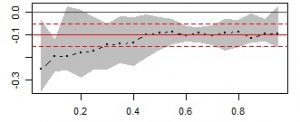Want to share your content on R-bloggers? click here if you have a blog, or here if you don't.The standard OLS (Ordinary Least Squares) model explains the relationship between independent variables and the conditional mean of the dependent variable. In contrast, quantile regression models this relationship for different quantiles of the dependent variable.
In this exercise set we will use the `quantreg` package (package description: here) to implement quantile regression in R.

Answers to the exercises are available here.

Exercise 1
Load the `quantreg` package and the `barro` dataset (Barro and Lee, 1994). This has data on GDP growth rates for various countries.
Next, summarize the data.

Exercise 2
The dependent variable is `y.net` (Annual change per capita GDP). The remaining variables will be used to explain `y.net`. It is easier to combine variables using `cbind` before applying regression techniques. Combine variables so that we can write `Y ~ X`.

Exercise 3
Regress `y.net` on the independent variables using OLS. We will use this result as benchmark for comparison.

Exercise 4
Using the `rq` function, estimate the model at the median `y.net`. Compare results from exercise-3.

Learn more about Model Evaluation in the online course Regression Machine Learning with R. In this course you will learn how to:

• Avoid model over-fitting using cross-validation for optimal parameter selection
• Explore maximum margin methods such as best penalty of error term support vector machines with linear and non-linear kernels.
• And much more

Exercise 5
Estimate the model for the first and third quartiles and compare results.

Exercise 6
Using a single command estimate the model for 10 equally spaced deciles of `y.net`.

Exercise 7
`quantreg` package also offers shrinkage estimators to determine which variables play the most important role in predicting `y.net`. Estimate the model with LASSO based quantile regression at the median level with lambda=0.5.

Exercise 8
Quantile plots are most useful for interpreting results. To do that we need to define the sequence of percentiles. Use the `seq` function to define the sequence of percentiles from 5% to 95% with a jump of 5%.

Exercise 9
Use the result from exercise-8 to plot the graphs. Note that the red line is the OLS estimate bounded by the dotted lines which represent confidence intervals.

Exercise 10
Using results from exercise-5, test whether coefficients are significantly different for the first and third quartile based regressions.